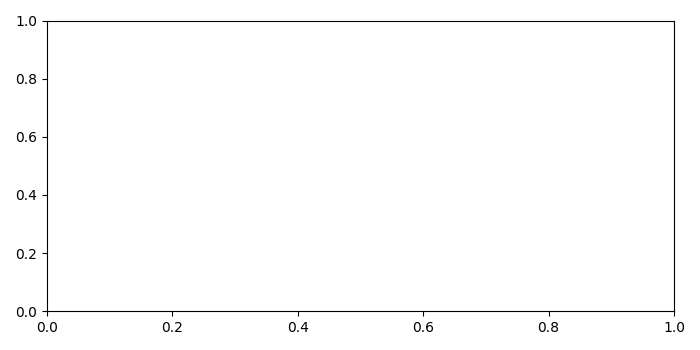# How do I close all the open pyplot windows (Matplotlib)?

plt.figure().close(): Close a figure window.

close() by itself closes the current figure

close(h), where h is a Figure instance, closes that figure

close(num) closes the figure with number=num

close(name), where name is a string, closes the figure with that label

close('all') closes all the figure windows

## Example

from matplotlib import pyplot as plt
fig = plt.figure()
plt.close()